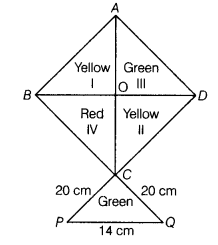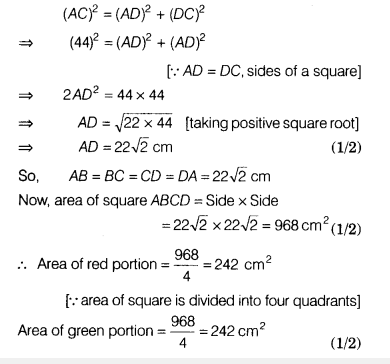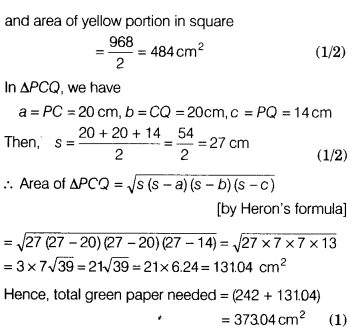# How much paper of each shade is needed to make kite given in figure

How much paper of each shade is needed to make kite given in figure, in which ABCD is a square with diagonal 44 cm?We know that all the sides of a square are equal.
AB = BC = CD = DA
In ∆ACD, we have
AC = 44 cm, ZD = 90°

Using Pythagoras theorem,